# HashMap

## 二、概念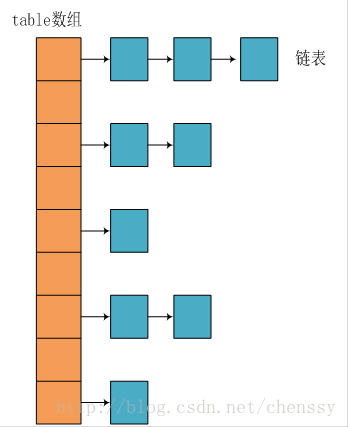Hashmap中获取元素时的主要流程步骤为，首先对key值进行hash算法得出hash值即哈希桶中的索引值，再找到对应的hash桶。如果存在，则通过（拉链法）链表从前往后比较value值是否相等，直到找到元素或下个节点为null时。

1. hash算法

//这是根据key值获取value值的方法
public V get(Object key) {
Node<K,V> e;
//先调用hash(key)
return (e = getNode(hash(key), key)) == null ? null : e.value;
}

//Hash算法如下
static final int hash(Object key) {
int h;
//第一步：先获取key中的hashCode值
return (key == null) ? 0 : (h = key.hashCode()) ^ (h >>> 16);
//第二步 再与hashcode向左移16位的值进行抑或
}
final Node<K,V> getNode(int hash, Object key) {
Node<K,V>[] tab; Node<K,V> first, e; int n; K k;
if ((tab = table) != null && (n = tab.length) > 0 &&
//第三步 n是指数组长度，hash与(n-1)进行&与运算
(first = tab[(n - 1) & hash]) != null) {
//省略
...
...
}
return null;
}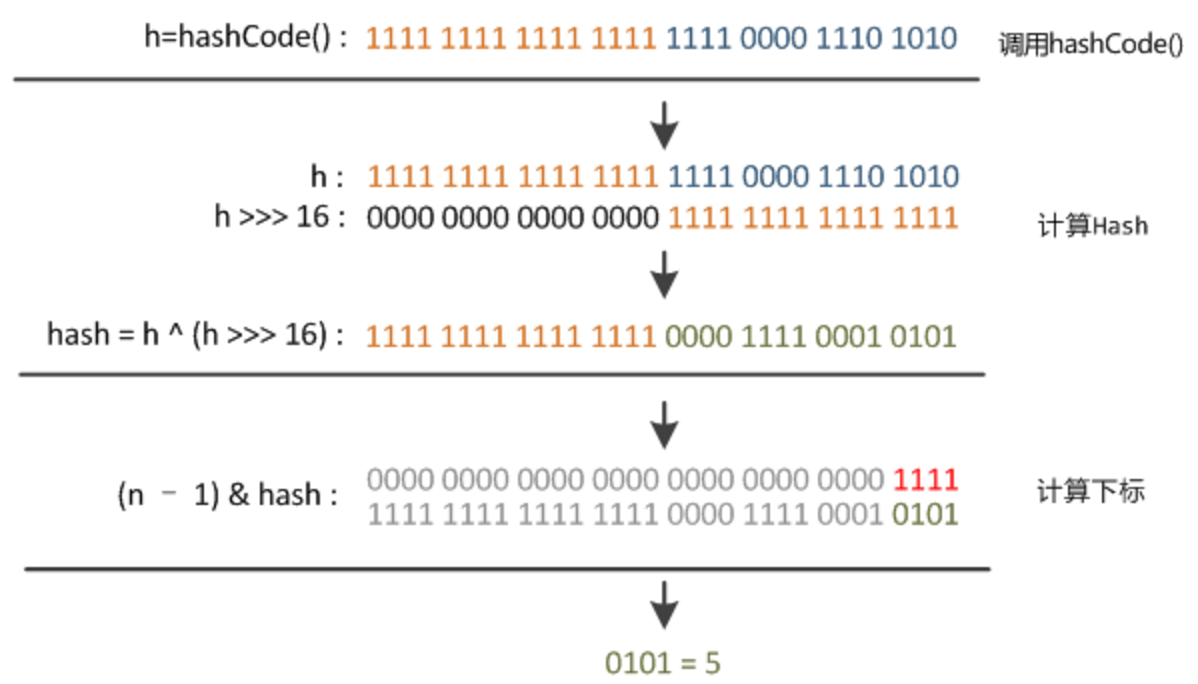2、get方法，查询元素

final Node<K,V> getNode(int hash, Object key) {
Node<K,V>[] tab; Node<K,V> first, e; int n; K k;
//如果表为null或长度为0，或者经过hash算法后得到的哈希桶为null，则直接返回null
if ((tab = table) != null && (n = tab.length) > 0 &&
(first = tab[(n - 1) & hash]) != null) {
//如果链表中的第一个节点元素相等则直接返回该Node
if (first.hash == hash && // always check first node
((k = first.key) == key || (key != null && key.equals(k))))
return first;
//第二个节点不为空时继续往后找
if ((e = first.next) != null) {
//判断是否为红黑树，是则交给红黑树去查找
if (first instanceof TreeNode)
return ((TreeNode<K,V>)first).getTreeNode(hash, key);
//否则循环链表找到对应相等的元素，直到找到或下个节点为null
do {
if (e.hash == hash &&
((k = e.key) == key || (key != null && key.equals(k))))
return e;
} while ((e = e.next) != null);
}
}
return null;
}
3.put方法，增加

put方法是重点也是最复杂的操作。需要掌握在什么情况下要扩容（后面会讲一下是如何扩容的），满足什么条件下链表需要转成红黑树。下面是put方法中流程图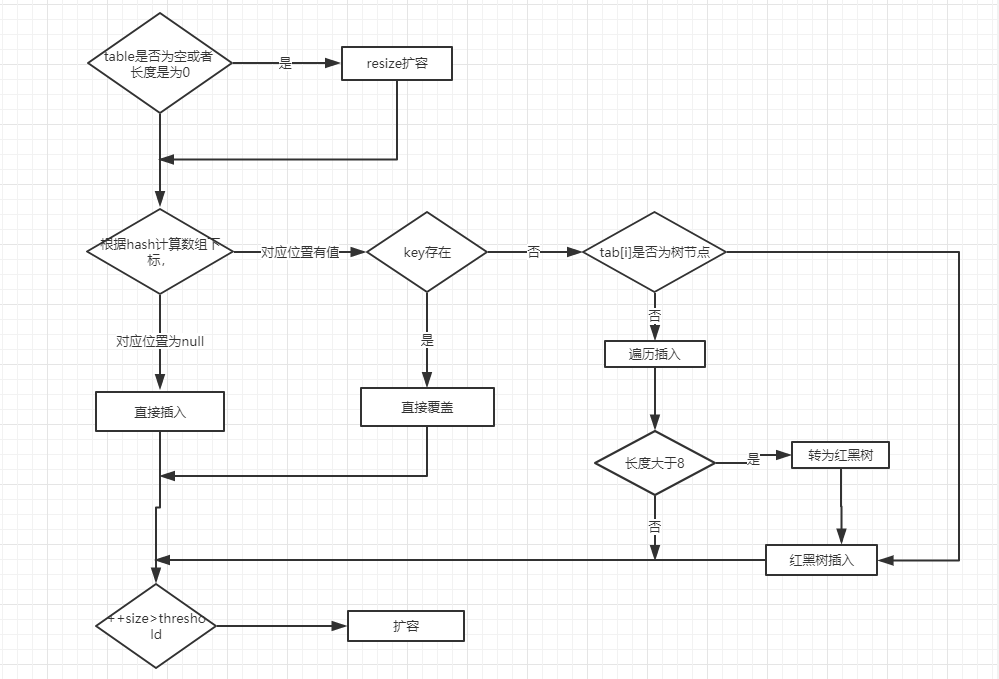final V putVal(int hash, K key, V value, boolean onlyIfAbsent,
boolean evict) {
Node<K,V>[] tab; Node<K,V> p; int n, i;
//table为null或length长度为0则扩容
if ((tab = table) == null || (n = tab.length) == 0)
n = (tab = resize()).length;
//如果哈希桶为null，则创建节点放在该桶内
if ((p = tab[i = (n - 1) & hash]) == null)
tab[i] = newNode(hash, key, value, null);
else {
Node<K,V> e; K k;
//如果桶内第一个元素hash相等，key相等，则更新此节点
if (p.hash == hash &&
((k = p.key) == key || (key != null && key.equals(k))))
e = p;
//判断是否为红黑树，若是则调用红黑树的putTreeVal
else if (p instanceof TreeNode)
e = ((TreeNode<K,V>)p).putTreeVal(this, tab, hash, key, value);
else {
//循环链表
for (int binCount = 0; ; ++binCount) {
//知道下个节点为null时
if ((e = p.next) == null) {
//增加节点
p.next = newNode(hash, key, value, null);
//如果桶内的节点是否大于8
if (binCount >= TREEIFY_THRESHOLD - 1) // -1 for 1st
//这个方法里还会判断总节点数大于64则会转换为红黑树
treeifyBin(tab, hash);
break;
}
//如果找到相等的节点则退出循环
if (e.hash == hash &&
((k = e.key) == key || (key != null && key.equals(k))))
break;
p = e;
}
}
//只有找到相等节点是e不为null
if (e != null) { // existing mapping for key
//更新节点为新的值
V oldValue = e.value;
if (!onlyIfAbsent || oldValue == null)
e.value = value;
afterNodeAccess(e);
return oldValue;
}
}
++modCount;
//最后判断增加后的个数是否大于阈值,大于则扩容
if (++size > threshold)
resize();
afterNodeInsertion(evict);
return null;
}
4.扩容

final Node<K,V>[] resize() {
Node<K,V>[] oldTab = table;
int oldCap = (oldTab == null) ? 0 : oldTab.length;
int oldThr = threshold;
int newCap, newThr = 0;
if (oldCap > 0) {
//如果容量大于了最大容量时，直接返回旧的table
if (oldCap >= MAXIMUM_CAPACITY) {
threshold = Integer.MAX_VALUE;
return oldTab;
}
//同时满足扩容两倍后小于最大容量和原先容量大于默认初始化的容量，对阈值增大两倍
else if ((newCap = oldCap << 1) < MAXIMUM_CAPACITY &&
oldCap >= DEFAULT_INITIAL_CAPACITY)
newThr = oldThr << 1; // double threshold
}

else if (oldThr > 0) // initial capacity was placed in threshold
newCap = oldThr;
else {               // zero initial threshold signifies using defaults
//默认初始化容量和阈值
newCap = DEFAULT_INITIAL_CAPACITY;
}
if (newThr == 0) {
float ft = (float)newCap * loadFactor;
newThr = (newCap < MAXIMUM_CAPACITY && ft < (float)MAXIMUM_CAPACITY ?
(int)ft : Integer.MAX_VALUE);
}
threshold = newThr;
@SuppressWarnings({"rawtypes","unchecked"})
Node<K,V>[] newTab = (Node<K,V>[])new Node[newCap];
table = newTab;
if (oldTab != null) {
//接下来对哈希桶的所有节点转移到新的哈希桶中
for (int j = 0; j < oldCap; ++j) {
Node<K,V> e;
//如果哈希桶为null，则不需任何操作
if ((e = oldTab[j]) != null) {
//将桶内的第一个节点赋值给e
//将原哈希桶置为null，让gc回收
oldTab[j] = null;
if (e.next == null)
//如果e的下个节点（即第二个节点）为null，则只需要将e进行转移到新的哈希桶中
newTab[e.hash & (newCap - 1)] = e;
else if (e instanceof TreeNode)
//如果哈希桶内的节点为红黑树，则交给TreeNode进行转移
((TreeNode<K,V>)e).split(this, newTab, j, oldCap);
else { // preserve order
//将桶内的转移到新的哈希桶内
//JDK1.8后将新的节点插在最后面

//下面就是1.8后的优化
//1.7是将哈希桶的所有元素进行hash算法后转移到新的哈希桶中
//而1.8后，则是利用哈希桶长度在扩容前后的区别，将桶内元素分为原先索引值和新的索引值（即原先索引值+原先容量）。这里不懂为什么，可以看下一段图文讲解。

//loTail是低位链表临时变量，记录上个节点并且让next指向当前节点
Node<K,V> loHead = null, loTail = null;
Node<K,V> hiHead = null, hiTail = null;
Node<K,V> next;
do {
//用于临时记录当前节点的next节点
next = e.next;
//e.hash & oldCap==0表示扩容前后对当前节点的索引值没有发生改变
if ((e.hash & oldCap) == 0) {
//loTail为null时，代表低位桶内无元素则记录头节点
if (loTail == null)
else
//将上个节点next指向当前节点
//即新的节点是插在链表的后面
loTail.next = e;
//将当前节点赋值给loTail
loTail = e;
}
else {
//跟上面的步骤是一样的。
if (hiTail == null)
else
hiTail.next = e;
hiTail = e;
}
//当next节点为null则退出循环
} while ((e = next) != null);
//如果低位链表记录不为null，则低位链表放到原index中
if (loTail != null) {
//将最后一个节点的next属性赋值为null
loTail.next = null;
}
//如果高位链表记录不为null，则高位链表放到新index中
if (hiTail != null) {
hiTail.next = null;
}
}
}
}
}
return newTab;
}
1.8对扩容方法进行了优化，经过rehash之后，元素的位置要么是在原位置，要么是在原位置再移动2次幂的位置。 看下图可以明白这句话的意思，n为table的长度，图（a）表示扩容前的key1和key2两种key确定索引位置的示例，图（b）表示扩容后key1和key2两种key确定索引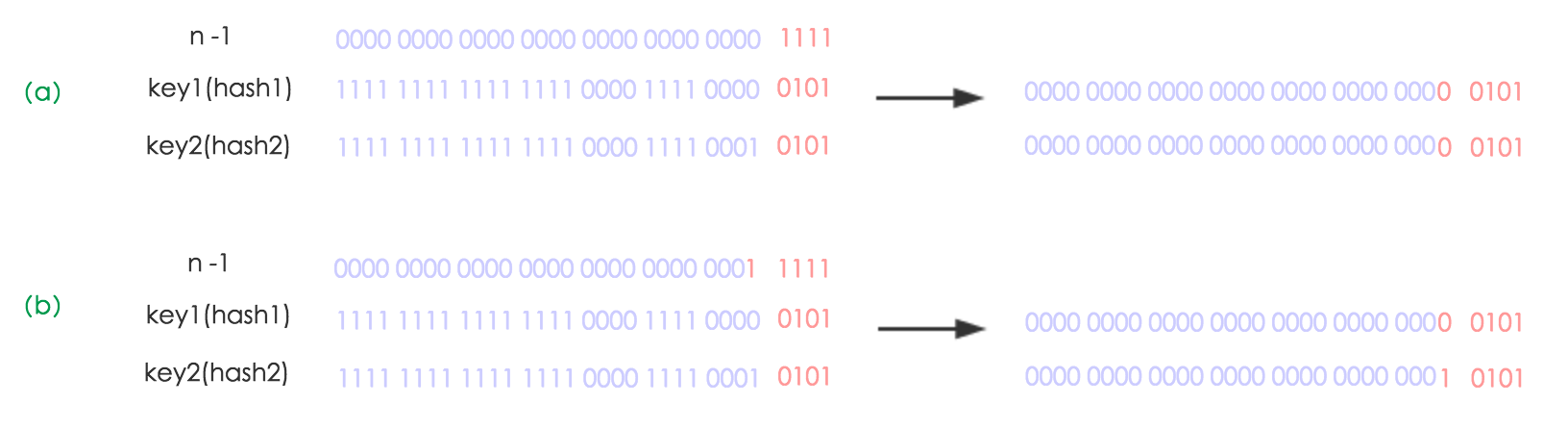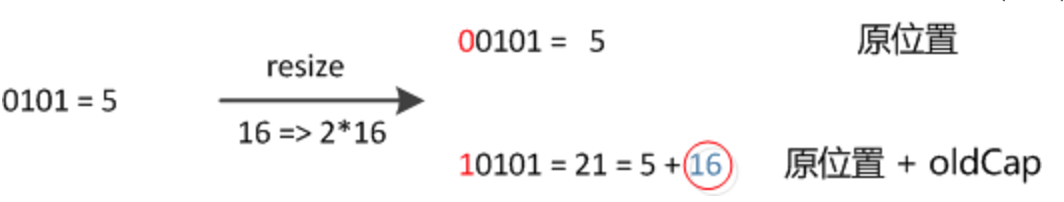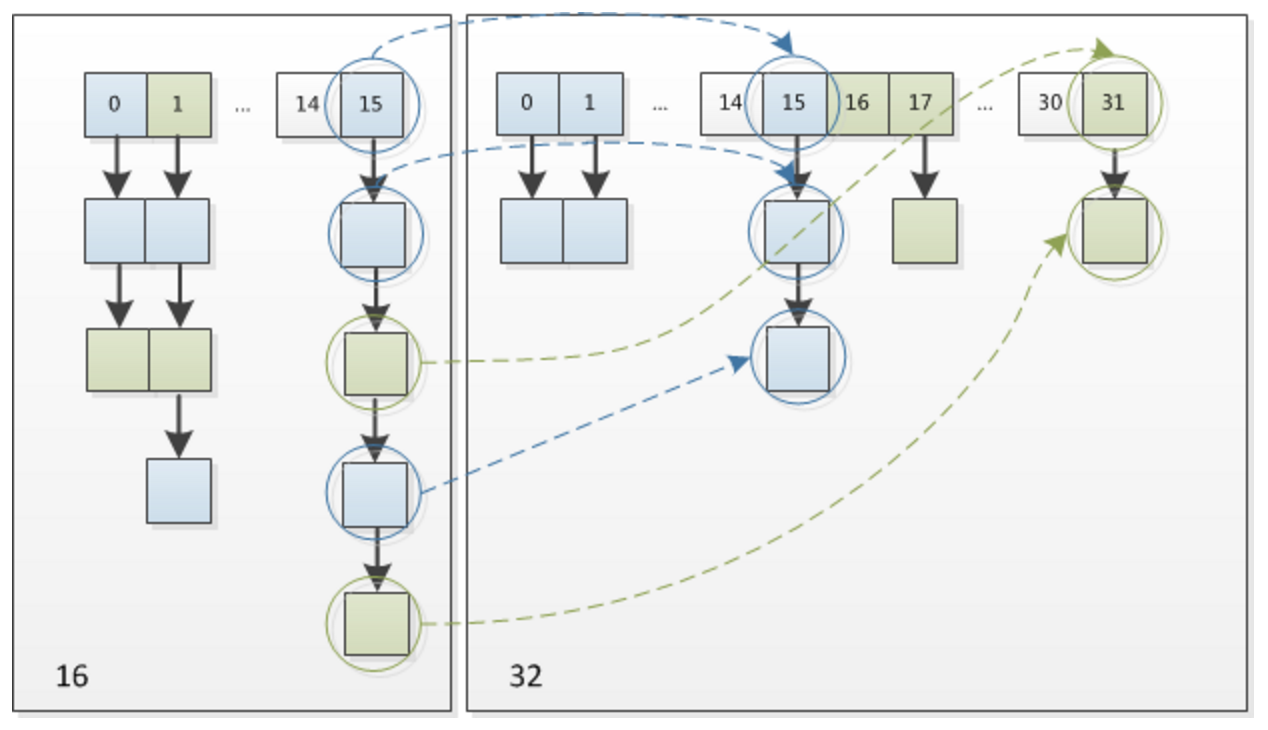————————————————

## 四、常见的面试题

1.HashMap底层是如何实现的？

1.8对扰动函数，扩容方法进行优化，并且增加了红黑树的数据结构。

2.HashMap 和 Hashtable 的区别

3.HashMap的长度为什么是2的倍数

4.Jdk1.8中满足什么条件后将链表转化成红黑树？

//putVal方法判断桶内元素是是否大于8
if (binCount >= TREEIFY_THRESHOLD - 1) // -1 for 1st
treeifyBin(tab, hash);
break;

//treeifyBin方法中判断长度是否大于最小红黑树容量64,小于则继续扩容，大于则转为红黑树
if (tab == null || (n = tab.length) < MIN_TREEIFY_CAPACITY)
resize();

©️2019 CSDN 皮肤主题: 大白 设计师: CSDN官方博客